# How to Select All Cells Whose Are in The Same Format in Excel

In excel we can mark texts from different cells in the same format, if we want to select all of them, we can select them one by one manually by holding Ctrl key, but it is very bothersome. This article will introduce you a quick way to select all cells in the same format at the same time, please see details below.

Precondition:

See screenshot below. Some texts like Lucy, Clair…, are marked in blue and bold. We can manually select them one by one, but if the range is expanded, manually select is annoying. So, we need another way to select them.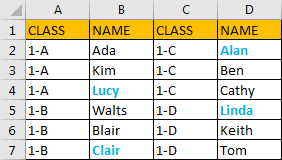## Method: Select All Cells Whose Are in The Same Format by Find Function in Excel

Step 1: Click Home in ribbon, then click Find & Select -> Find in Editing group. You can also click Ctrl+F to load and display Find and Replace dialog.Step 2: Click Options>> on Find and Replace dialog.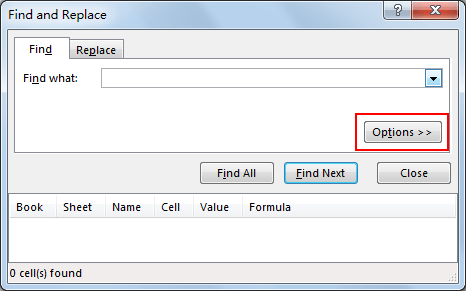Step 3: Click Format next to Find what preview box under Find tab.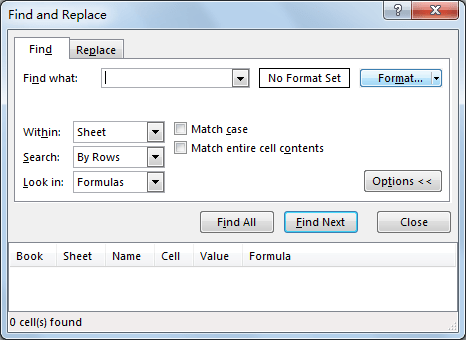Step 4: Click Arrow on Format button to load selections. Select ‘Choose Format From Cell…’.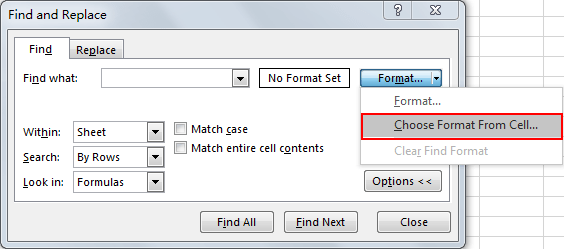Step 5: Select Lucy (or any other one in the same format), then in preview box text is displayed in selected format.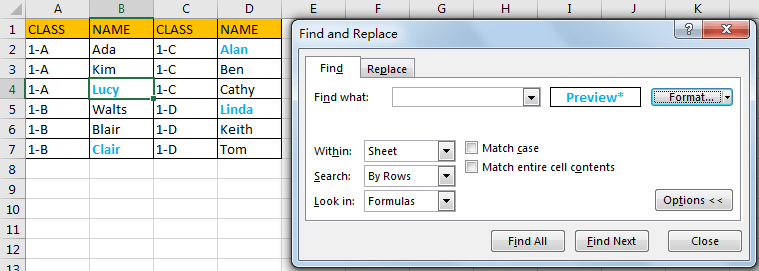Step 6: Click Find All button in the bottom. Verify that all cells have the same format are found.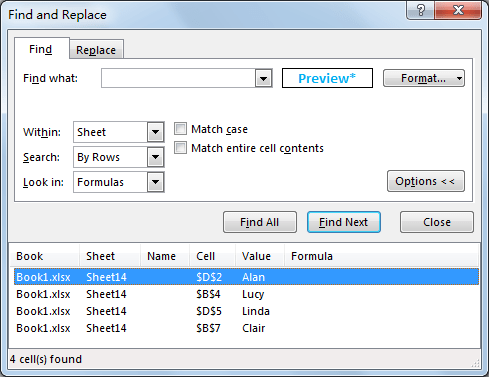Step 7: Press Ctrl key and click the last found cell, then all cells are selected. You can also press Ctrl+A to select all cells. Then you can do more operations for this group of cells.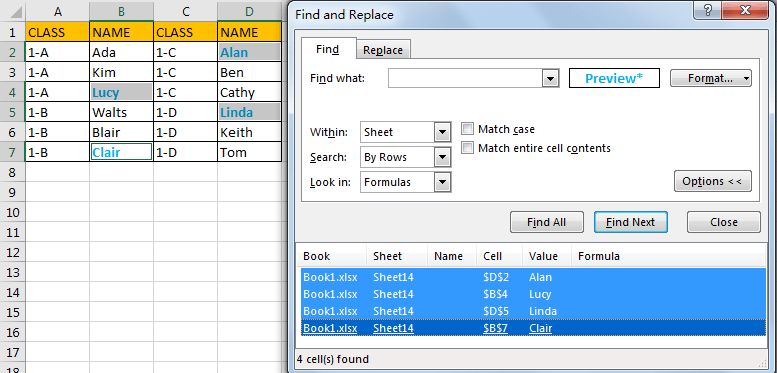Related Posts

Cap percentage values between 0 and 100

This article will talk about how to cap the percentage values between 0% and 100% in Microsoft Excel Spreadsheet or Google Sheets. If you are a newbie on Excel or google Sheets, you may be able to do this by ...

Calculate Cap Percentages to Specific Value

This article will talk about how to limit the cap percentage of a given amount to a given value in Microsoft Excel Spreadsheet or Google Sheets. If you are a newbie on Excel or google Sheets, you may be able ...

Calculate Win Loss Tie

Suppose you got a task to calculate the win, loss, and tie totals; what would you do? If you are new to Ms Excel and don't have enough experience with it, then you might do this task manually but let ...

Calculate Years Between Dates In Ms Excel

If you are an avid Ms Excel user, then you might have come across a task in which you needed to calculate the years between the dates; you might take it easy and do this task manually, which is also ...

Calculate Number of Hours between Two Times

Calculating the difference between two times might be a valuable statistic for subsequent computations or averages, whether you're producing a time sheet for staff or recording personal exercises. While Excel has a plethora of complex functions, including date and time ...

Calculate Loan Interest in Given Year

When you borrow money, you are supposed to repay it gradually. Lenders, on the other hand, want to be compensated for their services and the risk they incur by lending you money. That is, you will not just repay the ...

Calculate Interest Rate for Loan

The interest rate is the fee charged by a lender to a borrower and is expressed as a percentage of the principal—the lent amount. The interest rate on a loan is often expressed as an annual percentage rate, abbreviated as ...

Calculate Interest for Given Period

Using the IPMT function in Excel, we can compute the interest payment on any loan. This step-by-step tutorial will guide Excel users of all skill levels through the process to calculate interest for given period. Finally, the formula: =IPMT(B3/12,1,B5,-B2) The ...

How To Use Excel GCD Function

This post will guide you how to use Excel GCD function with syntax and examples in Microsoft excel. Description The Excel GCD function Returns the greatest common divisor of two or more integers. So you can use the GCD function ...

Calculate A Ratio From Two Numbers In Excel

In elementary mathematics, a ratio is a connection or comparison between two or more integers. For example, ratios are often expressed as ":" to demonstrate the relationship between two numbers. You would think that manually calculating a ratio from two ...

Sidebar